### Two Dimensional Solid Elements: Quadrilateral Elements

It is usually easier to discretize a domain by using triangular elements; however, when the domain to be discretized has a regular shape, quadrilateral elements can be used to offer a more regular displacement discretization, which, in some cases, might offer a better approximation to the displacement shape. The bilinear quadrilateral element offers a bilinear displacement approximation where the displacement within the element is assumed to vary bilinearly (linear in two directions within the element). This element, however, behaves in a stiff manner when it is used to model linear strains (bending strains) since, as will be shown in the derivation, it is not possible to model pure bending (pure linear normal strain components in one direction) without introducing additional shear strains (usually termed parasitic shear strains).

Consider the plane quadrilateral shown in Figure 7. The bilinear displacement function for this element is assumed to have the following form:

(1)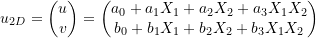where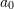,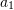,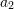,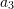,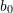,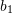,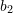, and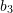are eight generalized degrees of freedom of the element. The displacement function in terms of the nodal degrees of freedom has the form: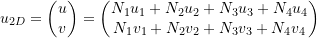There are two ways to find the shape functions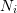. The first way is to replace the generalized degrees of freedom with the nodal degrees of freedom in the first equation. The multipliers of the nodal degrees of freedom would then be the shape function. The following mathematica code does that for you:

View Mathematica Code

Clear[a, b]
u = a0 + a1*X1 + a2*X2 + a3*X1*X2;
v = b0 + b1*X1 + b2*X2 + b3*X1*X2;
Coordinates = {{X1 -> -a, X2 -> -b}, {X1 -> a, X2 -> -b}, {X1 -> a, X2 -> b}, {X1 -> -a, X2 -> b}};
Eq1 = u /. Coordinates[];
Eq2 = u /. Coordinates[];
Eq3 = u /. Coordinates[];
Eq4 = u /. Coordinates[];
Eq5 = v /. Coordinates[];
Eq6 = v /. Coordinates[];
Eq7 = v /. Coordinates[];
Eq8 = v /. Coordinates[];
sol = Solve[{Eq1 == u1, Eq2 == u2, Eq3 == u3, Eq4 == u4, Eq5 == v1, Eq6 == v2, Eq7 == v3, Eq8 == v4}, {a0, a1, a2, a3, b0, b1, b2, b3}]
u = u /. sol[];
v = v /. sol[];
N1 = FullSimplify[Coefficient[u, u1]]
N1 = FullSimplify[Coefficient[v, v1]]
N2 = FullSimplify[Coefficient[u, u2]]
N2 = FullSimplify[Coefficient[v, v2]]
N3 = FullSimplify[Coefficient[u, u3]]
N3 = FullSimplify[Coefficient[v, v3]]
N4 = FullSimplify[Coefficient[u, u4]]
N4 = FullSimplify[Coefficient[v, v4]]

View Python Code

import sympy as sp
from sympy import *
a,a0,a1,a2,a3,b,b0,b1,b2,b3,X1,X2,u1,u2,u3,u4,v1,v2,v3,v4 = symbols("a a_0 a_1 a_2 a_3 b b_0 b_1 b_2 b_3 X_1 X_2 u_1 u_2 u_3 u_4 v_1 v_2 v_3 v_4")
u = a0+a1*X1+a2*X2+a3*X1*X2
v = b0+b1*X1+b2*X2+b3*X1*X2
Coordinates = [{X1:-a,X2:-b},{X1:a,X2:-b},{X1:a,X2:b}, {X1:-a, X2:b}]
display("Coordinates: ",Coordinates)
Eq1 = u.subs(Coordinates)
Eq2 = u.subs(Coordinates)
Eq3 = u.subs(Coordinates)
Eq4 = u.subs(Coordinates)
Eq5 = v.subs(Coordinates)
Eq6 = v.subs(Coordinates)
Eq7 = v.subs(Coordinates)
Eq8 = v.subs(Coordinates)
s = solve((Eq1-u1,Eq2-u2,Eq3-u3,Eq4-u4,Eq5-v1,Eq6-v2, Eq7-v3, Eq8-v4),
(a0,a1,a2,a3,b0,b1,b2,b3))
display("Solve: ",s)
u = u.subs(s).expand()
v = v.subs(s).expand()
display("u =",u,"v =",v)
N1_u = u.coeff(u1)
N1_v = v.coeff(v1)
N2_u = u.coeff(u2)
N2_v = v.coeff(v2)
N3_u = u.coeff(u3)
N3_v = v.coeff(v3)
N4_u = u.coeff(u4)
N4_v = v.coeff(v4)
display("N1u1 =",N1_u,"N1v1 =",N1_v,"N2u2 =",N2_u,
"N2v2 =",N2_v,"N3u3 =",N3_u,"N3v3 =",N3_v,
"N4u4=",N4_u,"N4v4 =",N4_v)


An alternate way is directly by realizing that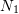has to satisfy that the condition that at node 1,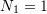, and is equal to zero on the lines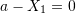and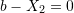. The same can be applied for N_2 and N_3. These result in the following expressions for the shape functions: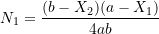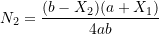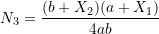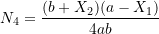The distribution of the shape functions on the element are illustrated in Figure 8.

The strain associated with the assumed displacement field has the following form: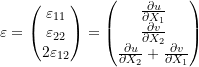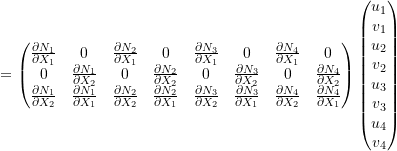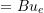Where, the explicit representation of the matrix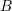is: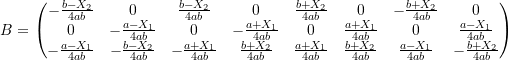##### Shear Locking

A quick glance at the matrixshows that the bilinear quadrilateral can model bending (linear strains) since the strains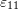and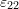contain linear expressions in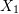and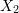. However, it will be shown here that to model linear strains, the element predicts an associated shear strain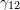as well (termed parasitic shear strains). For simplicity, we will calculate the strains based on Equation 1. The strains have the form: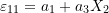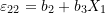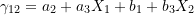If we now assume pure bending state of strain with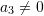. Then, the shear strain cannot be equal to zero sinceappears in the expression for! This behaviour is termed shear locking and causes the element to be too stiff under pure bending.

##### Stiffness Matrix

The constitutive relationship of plane linear elastic materials is defined using a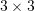matrix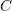that depends on whether the material is in a plane strain or a plane stress state. For the sake of illustration, the plane strain state is chosen here, the material constitutive relationship matrix in that case is: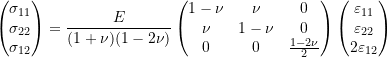The stiffness matrix of the linear strain triangle can be evaluated using the following integral (assuming a constant thickness: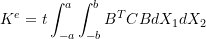The stiffness matrix has the dimensions of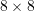, and the following Mathematica code can be utilized to view its components:

View Mathematica Code

Shapefun=Table[0,{i,1,4}];
Shapefun[]=(b-x2)(a-x1)/4/a/b;
Shapefun[]=(b-x2)(a+x1)/4/a/b;
Shapefun[]=(b+x2)(a+x1)/4/a/b;
Shapefun[]=(b+x2)(a-x1)/4/a/b;
B=Table[0,{i,1,3},{j,1,8}];
Do[B[[1,2i-1]]=B[[3,2i]]=D[Shapefun[[i]],x1];B[[2,2i]]=B[[3,2i-1]]=D[Shapefun[[i]],x2],{i,1,4}];
Cc=Ee/(1+nu)*{{(1-nu)/(1-2nu),nu/(1-2nu),0},{(nu)/(1-2nu),(1-nu)/(1-2nu),0},{0,0,1/2}};
Kbeforeintegration=t*FullSimplify[Transpose[B].Cc.B];
K=Integrate[Kbeforeintegration,{x2,-b,b},{x1,-a,a}];
K//MatrixForm

View Python Code

import sympy as sp
from sympy import *
a, b, x1, x2, nu, E, t = symbols("a b x_1 x_2 nu E t")
Shapefun = Matrix([(b-x2)*(a-x1)/4/a/b,(b-x2)*(a+x1)/4/a/b,(b+x2)*(a+x1)/4/a/b,(b+x2)*(a-x1)/4/a/b])
B = zeros(3,8)
for i in range(4):
B[0,2*i] = B[2,2*i+1] = Shapefun[i].diff(x1)
B[1,2*i+1] = B[2,2*i] = Shapefun[i].diff(x2)
display("B:", B)
Cc = E/(1 + nu)*Matrix([[(1-nu)/(1-2*nu),nu/(1-2*nu),0],
[nu/(1-2*nu),(1-nu)/(1-2*nu), 0],
[0, 0, 1/2]])
KBeforeintegration = t * B.transpose()*Cc*B
K = Matrix([[simplify(integrate(KBeforeintegration[i,j],(x1,-a,a),(x2,-b,b)))for i in range (8)] for j in range (8)])
display("K: ", K)


The nodal loads due to distributed body forces and traction forces on the boundaries are equally distributed among the nodes. The same procedure followed for the triangular elements can be repeated to obtain the distribution shown in Figure 9. The following Mathematica code can be utilized for the calculations producing the distributions in Figure 9.

View Mathematica Code

Shapefun=Table[0,{i,1,4}];
Shapefun[]=(b-x2)(a-x1)/4/a/b;
Shapefun[]=(b-x2)(a+x1)/4/a/b;
Shapefun[]=(b+x2)(a+x1)/4/a/b;
Shapefun[]=(b+x2)(a-x1)/4/a/b;
Nn=Table[0,{i,1,2},{j,1,8}];
Do[Nn[[1,2i-1]]=Nn[[2,2i]]=Shapefun[[i]],{i,1,4}];
tn={t1,t2};
rb={rb1,rb2};
fetraction=Integrate[(Transpose[Nn].tn/.x1->-a),{x2,-b,b}]//MatrixForm
febodyforces=Integrate[(Transpose[Nn].rb),{x2,-b,b},{x1,-a,a}]//MatrixForm

View Python Code

import sympy as sp
from sympy import *
a, b, x1, x2, t1, t2, rb1, rb2 = symbols("a b x_1 x_2 t_1 t_2 rb_1 rb_2")
Shapefun = Matrix([(b-x2)*(a-x1)/4/a/b,(b-x2)*(a+x1)/4/a/b,(b+x2)*(a+x1)/4/a/b,(b+x2)*(a-x1)/4/a/b])
Nn= zeros(2,8)
for i in range(4):
Nn[0,2*i] = Nn[1,2*i+1] = Shapefun[i]
display("Nn:", Nn)
tn = Matrix([t1,t2])
rb = Matrix([rb1,rb2])
integrand1 = (Nn.transpose()*tn).subs(x1,-a)
integrand2 = (Nn.transpose()*rb)
fetraction = Matrix([simplify(integrate(integrand1[i],(x2,-b,b)))for i in range (8)])
febodyforces = Matrix([simplify(integrate(integrand2[i],(x1,-a,a),(x2,-b,b)))for i in range (8)])
display("fetraction: ", fetraction)
display("febodyforces: ", febodyforces)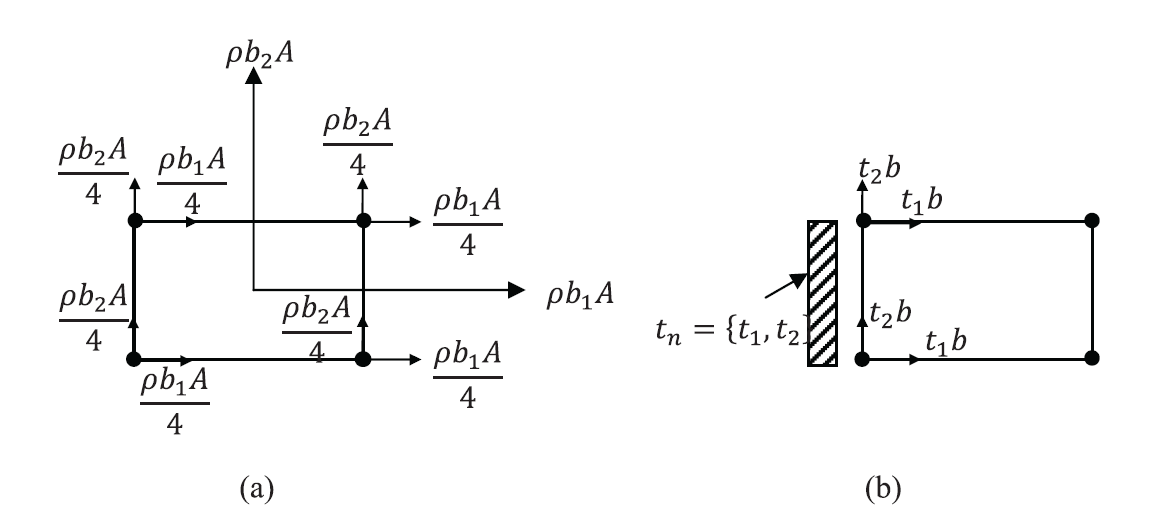Figure 9. Nodal forces in a bilinear quadrilateral element with a constant unit thickness due to (a) constant body forces vectors, (b) constant traction vector on one side.

By introducing four additional nodes in the mid-sides of a quadrilateral element, the displacement shape within the element can have a quadratic form, and the parasitic shear stiffness of the bilinear quadrilateral can be avoided. The quadratic quadrilateral offers such advantage with the price of higher computational time due to having additional degrees of freedom. Consider the plane quadrilateral shown in Figure 10. The trial displacement function has the following form: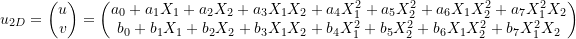where,,,,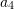,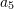,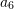,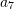,,,,,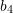,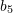,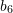and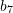are 16 generalized degrees of freedom of the element. The displacement function in terms of the nodal degrees of freedom has the form: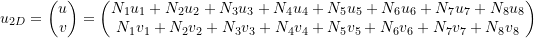Following the procedures shown in the previous sections, the shape functions can be shown to have the following forms: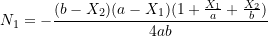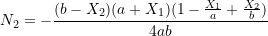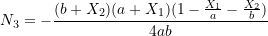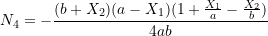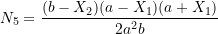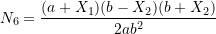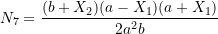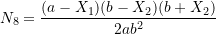The distribution of the shape functions on the element are illustrated in Figure 11.

##### Stiffness Matrix

The constitutive relationship of plane linear elastic materials is defined using amatrixthat depends on whether the material is in a plane strain or a plane stress state. For the sake of illustration, the plane strain state is chosen here, the material constitutive relationship matrix in that case is:The stiffness matrix of the linear strain triangle can be evaluated using the following integral (assuming a constant thickness:The stiffness matrix has the dimensions of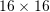, and the following Mathematica code can be utilized to view its components:

View Mathematica Code

Shapefun=Table[0,{i,1,8}];
Shapefun[]=(b-x2)(a-x1)/4/a/b*-(1+x1/a+x2/b);
Shapefun[]=(b-x2)(a+x1)/4/a/b*-(1-x1/a+x2/b);
Shapefun[]=(b+x2)(a+x1)/4/a/b*-(1-x1/a-x2/b);
Shapefun[]=(b+x2)(a-x1)/4/a/b*-(1+x1/a-x2/b);
Shapefun[]=(b-x2)(a-x1)(a+x1)/2/a^2/b;
Shapefun[]=(a+x1)(b-x2)(b+x2)/2/a/b^2;
Shapefun[]=(b+x2)(a-x1)(a+x1)/2/a^2/b;
Shapefun[]=(a-x1)(b-x2)(b+x2)/2/a/b^2;
B=Table[0,{i,1,3},{j,1,16}];
Do[B[[1,2i-1]]=B[[3,2i]]=D[Shapefun[[i]],x1];B[[2,2i]]=B[[3,2i-1]]=D[Shapefun[[i]],x2],{i,1,8}];
B//MatrixForm
Cc=Ee/(1+nu)*{{(1-nu)/(1-2nu),nu/(1-2nu),0},{(nu)/(1-2nu),(1-nu)/(1-2nu),0},{0,0,1/2}};
Kbeforeintegration=t*FullSimplify[Transpose[B].Cc.B];
K=Integrate[Kbeforeintegration,{x2,-b,b},{x1,-a,a}];
K//MatrixForm

View Python Code

import sympy as sp
from sympy import *
a, b, x1, x2, E, t, nu = symbols("a b x_1 x_2 E t nu")
Shapefun = Matrix([(b-x2)*(a-x1)/4/a/b*-(1+x1/a+x2/b),
(b-x2)*(a+x1)/4/a/b*-(1-x1/a+x2/b),
(b+x2)*(a+x1)/4/a/b*-(1-x1/a-x2/b),
(b+x2)*(a-x1)/4/a/b*-(1+x1/a-x2/b),
(b-x2)*(a-x1)*(a+x1)/2/a**2/b,
(a+x1)*(b-x2)*(b+x2)/2/a/b**2,
(b+x2)*(a-x1)*(a+x1)/2/a**2/b,
(a-x1)*(b-x2)*(b+x2)/2/a/b**2])
B = zeros(3,16)
for i in range(8):
B[0,2*i] = B[2,2*i+1] = Shapefun[i].diff(x1)
B[1,2*i+1] = B[2,2*i] = Shapefun[i].diff(x2)
display("B:", B)
Cc = E/(1 + nu)*Matrix([[(1-nu)/(1-2*nu),nu/(1-2*nu),0],
[nu/(1-2*nu),(1-nu)/(1-2*nu), 0],
[0, 0, 1/2]])
KBeforeintegration = t * B.transpose()*Cc*B
K = Matrix([[simplify(integrate(KBeforeintegration[i,j],(x1,-a,a),(x2,-b,b)))for i in range (16)] for j in range (16)])
display("K: ", K)

##### Nodal Forces

Assuming that the distributed body forces vector per unit mass is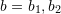, the mass density is 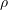, and the traction vector per unit area on the left side is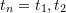, then, the lumped nodal forces due to the distributed body forces can be obtained as follows: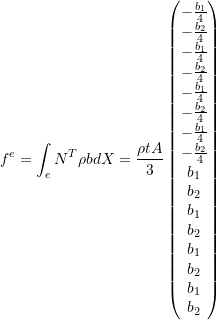The lumped nodal forces due to the distributed traction vector has the following form: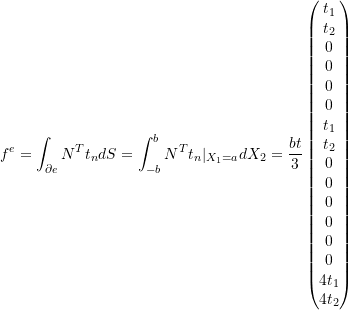The nodal loads due to a constant distributed body forces vector and the traction vector on the left side are shown in Figure 12. Notice the surprising result of having small forces applied on the corner nodes opposite in direction to the applied body forces.

View Mathematica Code

Shapefun=Table[0,{i,1,8}];
Shapefun[]=(b-x2)(a-x1)/4/a/b*-(1+x1/a+x2/b);
Shapefun[]=(b-x2)(a+x1)/4/a/b*-(1-x1/a+x2/b);
Shapefun[]=(b+x2)(a+x1)/4/a/b*-(1-x1/a-x2/b);
Shapefun[]=(b+x2)(a-x1)/4/a/b*-(1+x1/a-x2/b);
Shapefun[]=(b-x2)(a-x1)(a+x1)/2/a^2/b;
Shapefun[]=(a+x1)(b-x2)(b+x2)/2/a/b^2;
Shapefun[]=(b+x2)(a-x1)(a+x1)/2/a^2/b;
Shapefun[]=(a-x1)(b-x2)(b+x2)/2/a/b^2;
Nn=Table[0,{i,1,2},{j,1,16}];
Do[Nn[[1,2i-1]]=Nn[[2,2i]]=Shapefun[[i]],{i,1,8}];
tn={t1,t2};
rb={rb1,rb2};
fetraction=Integrate[(Transpose[Nn].tn/.x1->-a),{x2,-b,b}]//MatrixForm
febodyforces=Integrate[(Transpose[Nn].rb),{x2,-b,b},{x1,-a,a}]//MatrixForm

View Python Code

import sympy as sp
from sympy import *
a, b, x1, x2, t1, t2, rb1, rb2 = symbols("a b x_1 x_2 t_1 t_2 rb_1 rb_2")
Shapefun = Matrix([(b-x2)*(a-x1)/4/a/b*-(1+x1/a+x2/b),
(b-x2)*(a+x1)/4/a/b*-(1-x1/a+x2/b),
(b+x2)*(a+x1)/4/a/b*-(1-x1/a-x2/b),
(b+x2)*(a-x1)/4/a/b*-(1+x1/a-x2/b),
(b-x2)*(a-x1)*(a+x1)/2/a**2/b,
(a+x1)*(b-x2)*(b+x2)/2/a/b**2,
(b+x2)*(a-x1)*(a+x1)/2/a**2/b,
(a-x1)*(b-x2)*(b+x2)/2/a/b**2])
Nn = zeros(2,16)
for i in range(8):
Nn[0,2*i] = Nn[1,2*i+1] = Shapefun[i]
display("Nn:", Nn)
tn = Matrix([t1,t2])
rb = Matrix([rb1,rb2])
integrand1 = (Nn.transpose()*tn).subs(x1,-a)
integrand2 = (Nn.transpose()*rb)
fetraction = Matrix([simplify(integrate(integrand1[i],(x2,-b,b)))for i in range (16)])
febodyforces = Matrix([simplify(integrate(integrand2[i],(x1,-a,a),(x2,-b,b)))for i in range (16)])
display("fetraction: ", fetraction)
display("febodyforces: ", febodyforces)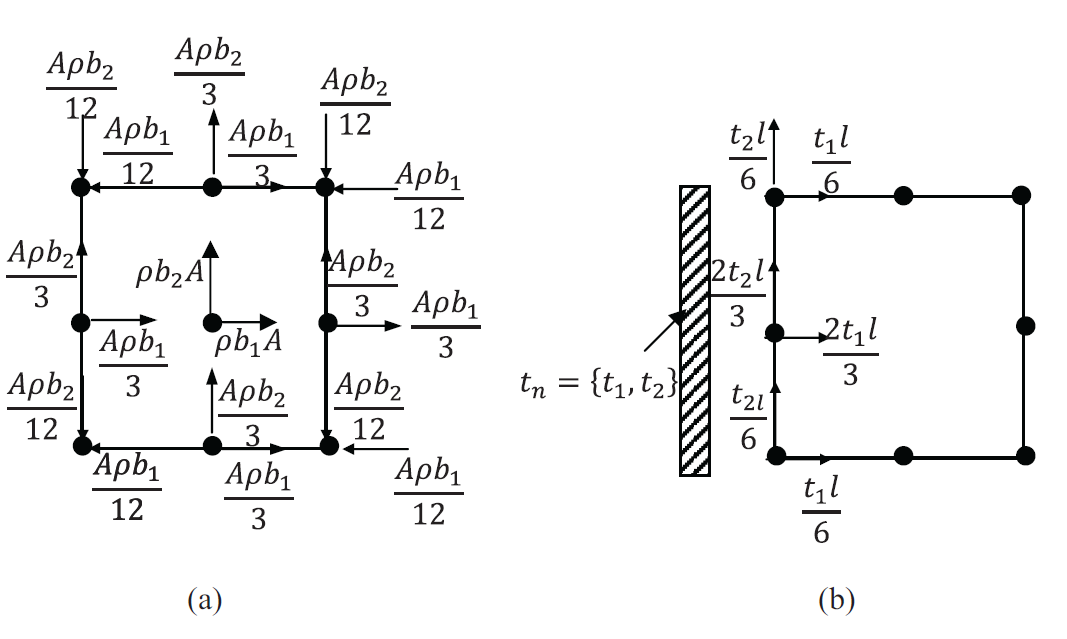Figure 12. Nodal forces in a quadratic quadrilateral element with a constant unit thickness due to (a) constant body forces vectors, (b) constant traction vector on one side. and .